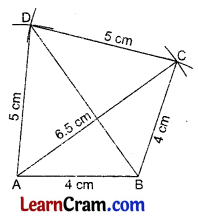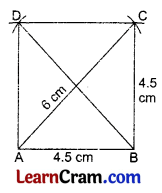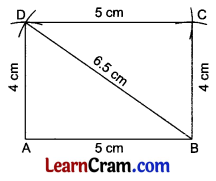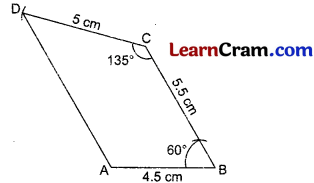# DAV Class 8 Maths Chapter 12 Worksheet 1 Solutions

The DAV Maths Class 8 Solutions and DAV Class 8 Maths Chapter 12 Worksheet 1 Solutions of Construction of Quadrilaterals offer comprehensive answers to textbook questions.

## DAV Class 8 Maths Ch 12 WS 1 Solutions

Question 1.
Construct a quadrilateral ABCD in which $$\overline{\mathrm{AB}}$$ = 4 cm, $$\overline{\mathrm{BC}}$$ = 5 cm, $$\overline{\mathrm{CD}}$$ = 4.5 cm, $$\overline{\mathrm{AD}}$$ = 5.5 cm and diagonal $$\overline{\mathrm{AC}}$$ = 7.5 cm. Measure diagonal $$\overline{\mathrm{BD}}$$.
Solution:
Steps of Construction:
1. Draw AB = 4 cm.
2. Draw arcs from A and B with radii 7.5 cm and 5 cm respectively which meet at C.
3. Join $$\overline{\mathrm{AC}}$$ and $$\overline{\mathrm{BC}}$$.
4. Draw two arcs from A and C with radii 5.5 cm and 4.5 cm respectively to meet at D.
5. Join $$\overline{\mathrm{AD}}$$ and $$\overline{\mathrm{CD}}$$ and $$\overline{\mathrm{BD}}$$.
6. ABCD is the required quadrilateral and $$\overline{\mathrm{BD}}$$ = 6 cm.Question 2.
Construct a quadrilateral PQRS in which sides $$\overline{\mathrm{PQ}}$$ = 3 cm, $$\overline{\mathrm{QR}}$$ = 4 cm, $$\overline{\mathrm{RS}}$$ = 3.5 cm,
$$\overline{\mathrm{SP}}$$ = 4 cm and diagonal $$\overline{\mathrm{PR}}$$ = 5 cm. Measure ∠PQR using a protractor.
Solution:
Steps of Construction:
1. Draw $$\overline{\mathrm{PQ}}$$ = 3 cm.
2. Draw arcs from P and Q with radii 5 cm and 4 cm respectively to meet at R.
3 Join $$\overline{\mathrm{PR}}$$ and $$\overline{\mathrm{QR}}$$.
4. Draw arcs from P and R with radii 4 cm and 3.5 cm respectively to meet at S.
5. Join PS and RS.
6. PQRS is the required quadrilateral.
7. ∠PQR = 90°.Question 3.
Construct a quadrilateral ABCD in which sides $$\overline{\mathrm{AB}}$$ = $$\overline{\mathrm{BC}}$$ = 4 cm, $$\overline{\mathrm{CD}}$$ = $$\overline{\mathrm{AD}}$$ = 5 cm and diagonal $$\overline{\mathrm{AC}}$$ = 6.5 cm. Draw diagonal $$\overline{\mathrm{BD}}$$ and measure it.
Solution:
Steps of Construction:
1. Draw $$\overline{\mathrm{AB}}$$ = 4 cm.
2. Draw arcs from A and B with radii 6.5 cm and 4 cm respectively to meet at C.
3. Join $$\overline{\mathrm{AC}}$$ and $$\overline{\mathrm{BC}}$$.
4. Draw arcs from A and C with a radius 5 cm each respectively to meet at D.
5. Join $$\overline{\mathrm{AD}}$$ and $$\overline{\mathrm{CD}}$$.
6. ABCD is the required quadrilateral.
7. Join $$\overline{\mathrm{BD}}$$ which is equal to 6.5 cm.Question 4.
Construct a quadrilateral ABCD in which each side is 4.5 cm and one diagonal $$\overline{\mathrm{AC}}$$ = 6 cm. Measure $$\overline{\mathrm{BD}}$$.
Solution:
Steps of Construction:
1. Draw $$\overline{\mathrm{AB}}$$ = 4.5 cm.
2. Draw arcs from A and B with radii 6 cm and 4.5 cm respectively to meet at C.
3. Draw two arcs from A and C with a radius of 4.5 cm each to meet at D.
4. Join $$\overline{\mathrm{AD}}$$ and $$\overline{\mathrm{DC}}$$.
5. ABCD is the required quadrilateral.
6. Join $$\overline{\mathrm{BD}}$$ which is equal to 6 cm.Question 5.
Construct a quadrilateral ABCD in which $$\overline{\mathrm{AB}}$$ = 4 cm, $$\overline{\mathrm{BC}}$$ = 3 cm. $$\overline{\mathrm{CD}}$$ = 4 cm, $$\overline{\mathrm{AD}}$$ = 3 cm and diagonal $$\overline{\mathrm{AC}}$$ = 5 cm. Measure diagonal $$\overline{\mathrm{BD}}$$. Is it equal to $$\overline{\mathrm{AC}}$$? Measure ∠A.
Solution:
Steps of Construction:
1. Draw $$\overline{\mathrm{AB}}$$ = 4 cm.
2. Draw arcs from A and B with radii 5 cm and 3 cm respectively to meet at C.
3. Join $$\overline{\mathrm{AC}}$$ and $$\overline{\mathrm{BC}}$$.
4. Draw two arcs from A and C with radii 3 cm and 4 cm respectively to meet at D.
5. Join $$\overline{\mathrm{CD}}$$ and $$\overline{\mathrm{AD}}$$.
6. ABCD is the required quadrilateral.
7. Join $$\overline{\mathrm{BD}}$$ and $$\overline{\mathrm{BD}}$$ = 5 cm.
8. Yes $$\overline{\mathrm{AC}}$$ and $$\overline{\mathrm{BD}}$$ = 5 cm
9. ∠A = 90°.Question 6.
Construct a quadrilateral ABCD in which $$\overline{\mathrm{AB}}$$ = $$\overline{\mathrm{CD}}$$ = 5 cm, $$\overline{\mathrm{BC}}$$ = $$\overline{\mathrm{AD}}$$ = 4 cm and $$\overline{\mathrm{BD}}$$ = 6.5 cm.
Solution:
Steps of Construction:
1. Draw $$\overline{\mathrm{AB}}$$ = 5 cm.
2. Draw arcs from A and B with radii 4 cm and 6.5 cm respectively to meet at D.
3. Join $$\overline{\mathrm{AD}}$$ and $$\overline{\mathrm{BD}}$$.
4. Draw arcs from D and B with radii 5 cm and 4 cm respectively to meet at C.
5. Join $$\overline{\mathrm{BC}}$$ and $$\overline{\mathrm{DC}}$$.
6. ABCD is the required quadrilateral.### DAV Class 8 Maths Chapter 12 Worksheet 1 Notes

Construction of a triangle can be done if

• the measure of its three sides are given. Or
• the measure of two angles and one side are given. Or
• the measure of two sides and one angle are given. Or
• one side and the hypotenuse of a right triangle are given.

Construction of a quadrilateral can be done if the measure of 4 sides or 3 sides and one angle or 2 sides and 2 angles or one diagonal and 3 sides are given.

Example 1.
Construct a quadrilateral PQRS if $$\overline{\mathrm{PQ}}$$ = 3.5 cm, $$\overline{\mathrm{QR}}$$ = 4.5 cm, $$\overline{\mathrm{RS}}$$ = 5 cm,
$$\overline{\mathrm{PS}}$$ = 5.5 cm, $$\overline{\mathrm{PR}}$$ = 6 cm.
Solution:Steps of Construction:

1. Draw $$\overline{\mathrm{PR}}$$ = 6 cm.
2. Draw two arcs from P and R with radius 3.5 cm and 4.5 cm respectively to meet at Q.
3. Join $$\overline{\mathrm{PQ}}$$ and $$\overline{\mathrm{RQ}}$$.
4. Draw two arcs from P and R with radius 5.5 cm and 5 cm respectively to meet at S.
5. Join $$\overline{\mathrm{PS}}$$ and $$\overline{\mathrm{RS}}$$.
6.  □PQRS is the reauired quadrilateral.Example 2.
Construct a quadrilateral ABCD in which $$\overline{\mathrm{AB}}$$ = 4 cm, $$\overline{\mathrm{BC}}$$ = 5 cm, $$\overline{\mathrm{CD}}$$ = 4.5 cm and the diagonal $$\overline{\mathrm{AC}}$$ = 6.5 cm and $$\overline{\mathrm{BD}}$$ = 7.5 cm.
Solution:Steps of Construction:

1. Draw $$\overline{\mathrm{AB}}$$ = 4 cm.
2. Draw two arcs from B and A with radius 5 cm and 6.5 cm respectively to meet at C.
3. Join $$\overline{\mathrm{AB}}$$ and AC.
4. Draw two arcs from B and C with radius 7.5 cm and 4.5 cm respectively to meet at D.
5. Join $$\overline{\mathrm{BD}}$$ and $$\overline{\mathrm{CD}}$$ and $$\overline{\mathrm{AD}}$$.
6. □ABCD is the required quadrilateral.

Example 3.
Construct a quadrilateral PQRS in which $$\overline{\mathrm{PQ}}$$ = 3.5 cm, $$\overline{\mathrm{QR}}$$ = 5 cm, ∠P = 750, ∠Q = 90° and ∠R = 120°.
Solution:Steps of Construction:

1. Draw $$\overline{\mathrm{PQ}}$$ = 3.5 cm.
2. Draw the angles of 75° at P and 90° at Q.
3. Draw $$\overline{\mathrm{QR}}$$ = 5 cm
4. Draw an angle of 120° at R which meets the angle line of 75° at S.
5. □PQRS is the required quadrilateral.Example 4.
Construct a DABCD in which $$\overline{\mathrm{AB}}$$ = 4.5 cm, $$\overline{\mathrm{BC}}$$ = 5.5 cm. $$\overline{\mathrm{CD}}$$ = 5 cm. ∠B = 60° and ∠C = 135°.
Solution:Steps of Construction:

1. Draw $$\overline{\mathrm{AB}}$$ = 4.5 cm.
2. Draw an angle of 60° at B and cut $$\overline{\mathrm{BC}}$$ = 5.5 cm.
3. Draw an angle of 135° at C and cut $$\overline{\mathrm{CD}}$$ = 5 cm.
4. Join $$\overline{\mathrm{AD}}$$.
5. □ABCD is the required quadrilateral.